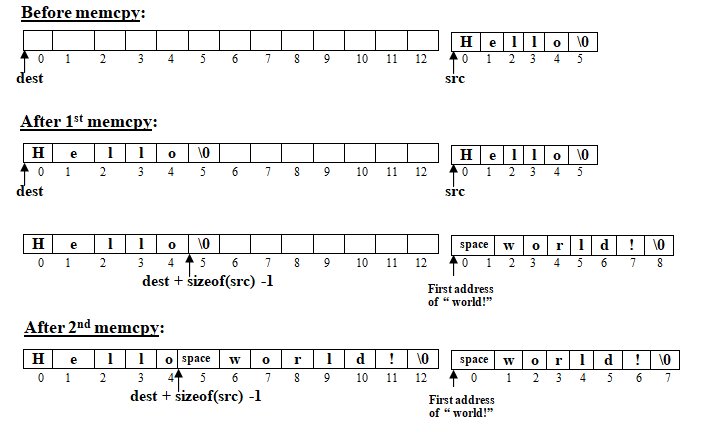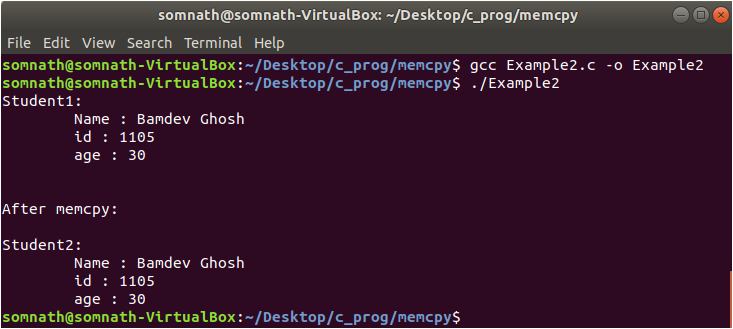13 C
Jaipur
Friday, January 15, 2021

# How to use memcpy function in C language? – Linux Hint

### Check benefits, full details here

In the C language memcpy() function is used to copy a block of memory from one location to another. In this article, we are going to discuss in detail how the memcpy() function is used. So, let’s get started.

### Syntax:

void * mempcpy (void *dest, const void *src, size_t size)

### Arguments:

The function takes 3 arguments:

1. dest :

This is a starting pointer of a memory block where the memory block pointed by src (2nd argument) will be copied. The pointer is declared as void, so any type of memory block can be copied.

2. src :

This is a starting pointer of the source memory block from where the memory block will be copied. The pointer is declared as void, so any type of memory block can be copied.

3. size :

This is the size of the memory block in bytes.

The value of the two pointer dest and src should be in such a way that two memory blocks do not overlap. The size of memory blocks of source and destination must be at least of size (3rd argument) bytes to avoid overlapping situations. If the two memory blocks are overlapped then the behavior of the memcpy() function is undefined. When there is a possibility of overlapping, you can use the memmove() library function where overlapping is well defined. memmove() function is slower compared to memcpy() function.

Due to the value of size, if the source or destination is accessed beyond their buffer length then the behavior of the memcpy() function is undefined.

The memcpy() function does not check to terminate ‘’ character.

### Return values:

This function returns the value of destination address dest. As the value of dest is already available so, it need not to store in any variable.

Examples:

//Example1.c

#include<stdio.h>
#include<string.h>

int main()
{
char src[] = “Hello”;
char dest;

memcpy(dest,src,6);

printf(“dest after first memcpy() => %sn,dest);

memcpy(dest+sizeof(src)1,” world!”,8);

printf(“dest after second memcpy() => %sn,dest);

return 0;
}

In Example1.c we have declared two-character array src and dest. The size of the src is 6 and the dest is 13. First, we copied 6 characters ‘H’, ‘e’, ‘l’, ‘l’, ‘o’, ‘’ from src to dest ( Line 11 ). In the second memcpy() function copied 8 characters ‘ ’, ‘w’, ‘o’, ‘r’, ‘l’, ‘d’, ‘!’, ‘’ to the dest after 5 characters ( Line 15 ). Pictorially we can represent this as follows://Example2.c

#include<stdio.h>
#include<string.h>

int main()
{
typedef struct student
{
char *name;
int id;
int age;
}std;

std student1; // Declare student1 of type std
std student2; // Declare student2 of type std

// Assigning the value of sudent1
student1.name = “Bamdev Ghosh”;
student1.id = 1105;
student1.age = 30;

printf(“Student1:ntName : %sntid : %dntage : %dn,student1.name,
student1.id,student1.age);

// Copy student1 to student2
memcpy(&student2, &student1, sizeof(student1));

printf(nnAfter memcpy:”);
printf(nnStudent2:ntName : %sntid : %dntage : %dn,
student2.name,student2.id,student2.age);

return 0;
}In Example2.c we have declared two structure student1 and student2 (Line 15 and 16). First, we initialize student1 (Line 19, 20, 21). After that, we use memcpy to copy data from student1 to student2.

### Conclusion:

In this article, we have learned how to use the memcpy function. We have seen that this function can be used for any type of memory block but this function has some limitations. So, you have to use this function carefully.